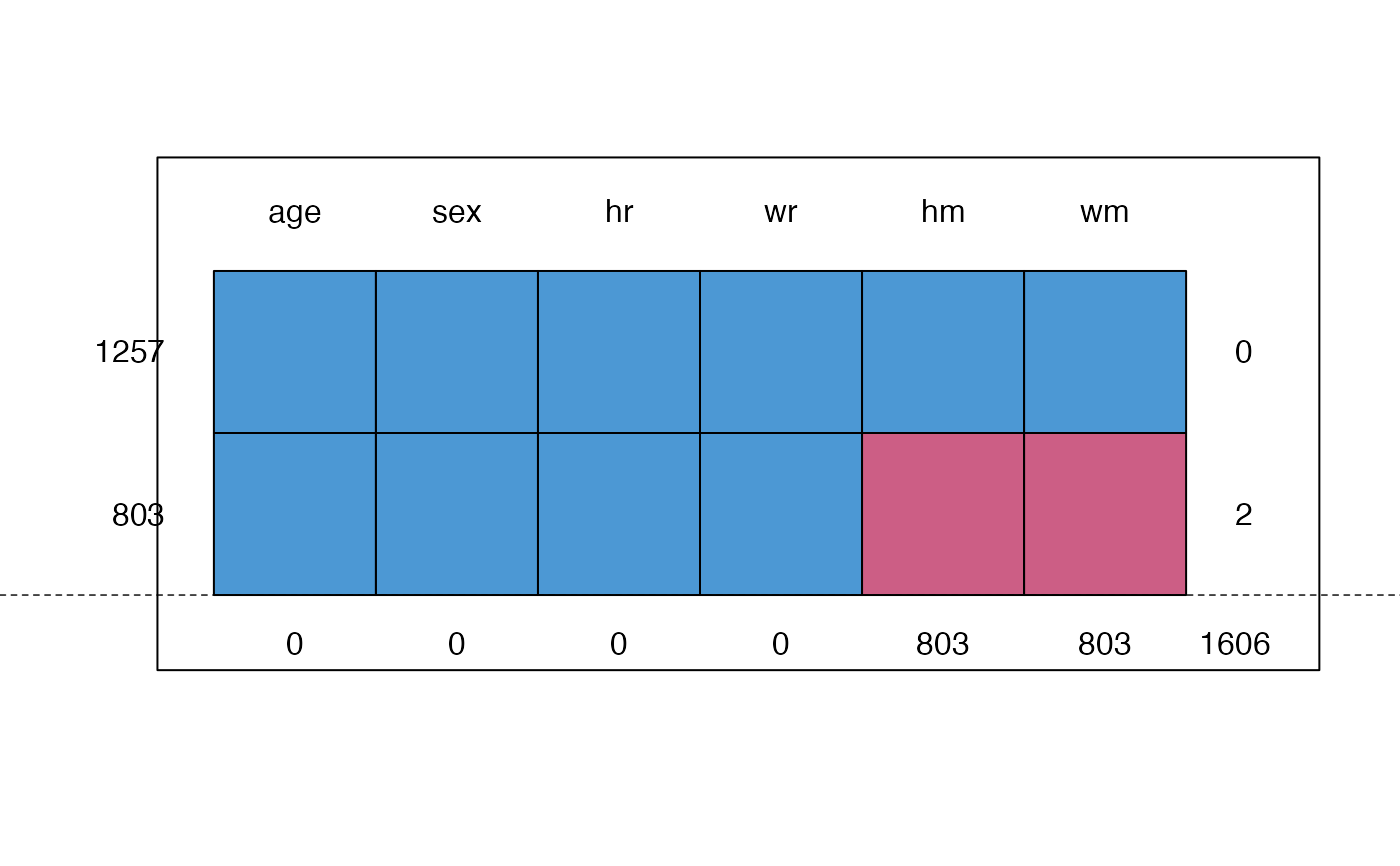Dataset containing height and weight data (measured, self-reported) from two studies.

## Format

A data frame with 2060 rows and 15 variables:

src

Study, either krul or mgg (factor)

id

Person identification number

pop

Population, all NL (factor)

age

Age of respondent in years

sex

Sex of respondent (factor)

hm

Height measured (cm)

wm

Weight measured (kg)

hr

Height reported (cm)

wr

Weight reported (kg)

prg

Pregnancy (factor), all Not pregnant

edu

Educational level (factor)

etn

Ethnicity (factor)

web

Obtained through web survey (factor)

bm

BMI measured (kg/m2)

br

BMI reported (kg/m2)

## Source

Krul, A., Daanen, H. A. M., Choi, H. (2010). Self-reported and measured weight, height and body mass index (BMI) in Italy, The Netherlands and North America. European Journal of Public Health, 21(4), 414-419.

Van Keulen, H.M.,, Chorus, A.M.J., Verheijden, M.W. (2011). Monitor Convenant Gezond Gewicht Nulmeting (determinanten van) beweeg- en eetgedrag van kinderen (4-11 jaar), jongeren (12-17 jaar) en volwassenen (18+ jaar). TNO/LS 2011.016. Leiden: TNO.

Van der Klauw, M., Van Keulen, H.M., Verheijden, M.W. (2011). Monitor Convenant Gezond Gewicht Beweeg- en eetgedrag van kinderen (4-11 jaar), jongeren (12-17 jaar) en volwassenen (18+ jaar) in 2010 en 2011. TNO/LS 2011.055. Leiden: TNO. (in Dutch)

Van Buuren, S. (2018). Flexible Imputation of Missing Data. Second Edition. Chapman & Hall/CRC. Boca Raton, FL.

## Details

This dataset combines two datasets: krul data (Krul, 2010) (1257 persons) and the mgg data (Van Keulen 2011; Van der Klauw 2011) (803 persons). The krul dataset contains height and weight (both measures and self-reported) from 1257 Dutch adults, whereas the mgg dataset contains self-reported height and weight for 803 Dutch adults. Section 7.3 in Van Buuren (2012) shows how the missing measured data can be imputed in the mgg data, so corrected prevalence estimates can be calculated.

## Examples

md.pattern(selfreport[, c("age", "sex", "hm", "hr", "wm", "wr")])
#>      age sex hr wr  hm  wm
#> 1257   1   1  1  1   1   1    0
#> 803    1   1  1  1   0   0    2
#>        0   0  0  0 803 803 1606

### FIMD Section 7.3.5 Application

bmi <- function(h, w) {
return(w / (h / 100)^2)
}
init <- mice(selfreport, maxit = 0)
#> Warning: Number of logged events: 2
meth <- init$meth meth["bm"] <- "~bmi(hm,wm)" pred <- init$pred
pred[, c("src", "id", "web", "bm", "br")] <- 0
imp <- mice(selfreport, pred = pred, meth = meth, seed = 66573, maxit = 2, m = 1)
#>
#>  iter imp variable
#>   1   1  hm  wm  edu  etn  bm
#> Error in bmi(hm, wm): could not find function "bmi"
## imp <- mice(selfreport, pred=pred, meth=meth, seed=66573, maxit=20, m=10)

### Like FIMD Figure 7.6

cd <- complete(imp, 1)
xy <- xy.coords(cd$bm, cd$br - cd$bm) #> Error in eval(expr, envir, enclos): object 'cd' not found plot(xy, col = mdc(2), xlab = "Measured BMI", ylab = "Reported - Measured BMI", xlim = c(17, 45), ylim = c(-5, 5), type = "n", lwd = 0.7 ) #> Error in eval(expr, envir, enclos): object 'xy' not found polygon(x = c(30, 20, 30), y = c(0, 10, 10), col = "grey95", border = NA) polygon(x = c(30, 40, 30), y = c(0, -10, -10), col = "grey95", border = NA) abline(0, 0, lty = 2, lwd = 0.7) idx <- cd$src == "krul"
xyc <- xy
xyc$x <- xy$x[idx]
xyc$y <- xy$y[idx]
xys <- xy
xys$x <- xy$x[!idx]
xys$y <- xy$y[!idx]